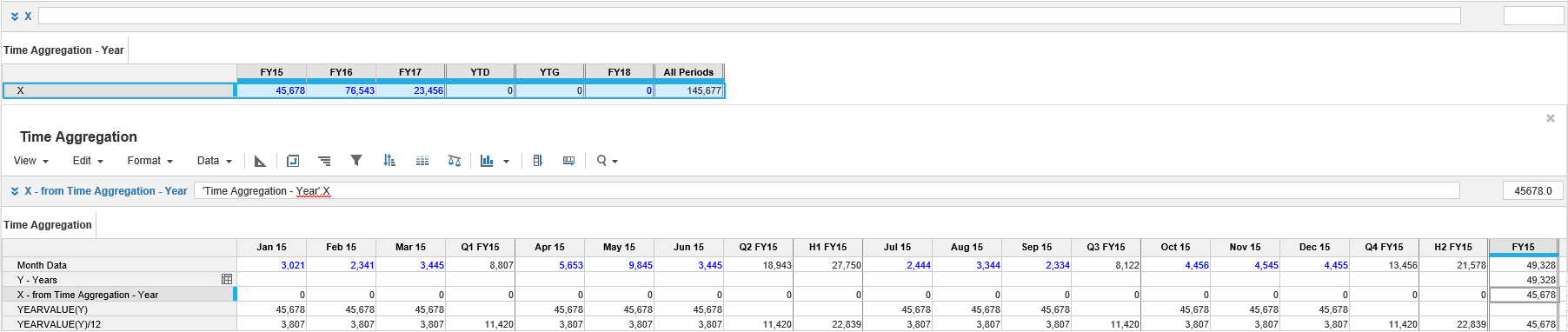Where two line items each have a different timescale, and one refers to the other in a formula, then days will aggregate into months, months aggregate into quarters, and quarters aggregate into years.

You can choose to skip levels: days could aggregate directly into years if required.

If line item X has a timescale of Months and line item Y has a timescale of Years, then

`Y=X`
will return the year total. However;
`X=Y`
will return nothing because Y contains no corresponding value for each month.

Formulas using the WEEKVALUE, MONTHVALUE, QUARTERVALUE, YEARVALUE or SELECT functions can be set up to return the result required.

For example, if X is in Months and Y is in Years:

 `Y - Years = Month Data` Result takes the relevant time total from Month Data, when Month Data has a more detailed timescale than Y - Years. `X - from X in Time Aggregation - Year` Shows a blank, unless Summary Method is set to Formula, in which case it will show the value Y in the year totals only (highlighted). `X = YEARVALUE(Y)` Will show the value of Y in each month. `X = YEARVALUE(Y)/12` Will allocate the value of Y evenly over months. `X = YEARVALUE(Y) * Seasonality%` Will allocate Y according to a seasonality percentage (not shown). `X = Y[SELECT:Time.FY11]` Will show the value of Y for a specific year (not shown).Remember! When you use any of the time functions, such as WEEKVALUE or MONTHVALUE, the value returned depends on the Summary method set for the source line item.